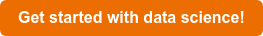# How is this related?

In this post, we'll take a look at how we can find out in what way data is structured or related.

Clustering or cluster analysis is a way of getting an idea about how data is structured or related.
For instance, when dealing with geographical data such as sightings of unidentified flying objects (UFOs) it may be interesting to see whether these sightings are clustered around certain points or how there are related.

The dataset we'll use in this post is a collection of UFO sightings from the last century. We'll use the latitude and longitude to see if the sightings are clustered around certain points.

` df = df.dropna(how='any') # remove null geo = df[['long','lat']].as_matrix(columns=None) # as matrix plt.scatter(geo[:, 0], geo[:, 1]); # scatter plot plt.show() # show plot plt.clf() # clear plot`When we simply plot latitude and longitude we can clearly see the sightings happen all around the world (which gives us a neat world map).

But how does this fit together?

A simple way of doing this is using K-Means clustering. With K-Means we decide on a number of centers around which our data is grouped.A downside to this method is that we have to manually decide on a valid number of clusters to run. Since we plotted the data above we can simply derive a quantity by looking at how our data is spread.

` kmeans = KMeans(n_clusters=4) # 4 clusters kmeans.fit(geo) # fit geo matrix y_kmeans = kmeans.predict(geo) # predict kmeans plt.scatter(geo[:, 0], geo[:, 1], c=y_kmeans, s=50, cmap='plasma') centers = kmeans.cluster_centers_ # cluster centers plt.scatter(centers[:, 0], centers[:, 1], c='black', s=150, alpha=0.5); # scatter plot plt.show() # show plot plt.clf() # clear plot`é

These four clusters are an example of how clustering can display relations between data, in this example they are (parts of) continents.
By calculating the centers of these clusters (or, more realistically, clusters on a lower level of detail) we could for instance compare them to landmarks or touristic areas such as memorials. This offers us the chance to further explore certain areas.

Get the code here, or get in touch if you want to dive deeper!